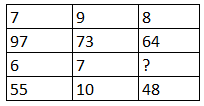Question 10

# Study the given pattern carefully and select the number that can replace the question mark (?)in it.Solution

As a forgiven question, the pattern followed in the form of the column

$$97 - 7 \times 6 = 97-42 = 55$$

$$73- 9\times 7 =73-63 = 10$$

Next $$64 - 8 \times ? = 48$$

$$\Rightarrow 64 - 8? = 48$$

$$\Rightarrow 8 ? = 16$$

$$\Rightarrow ? = 2$$ Ans